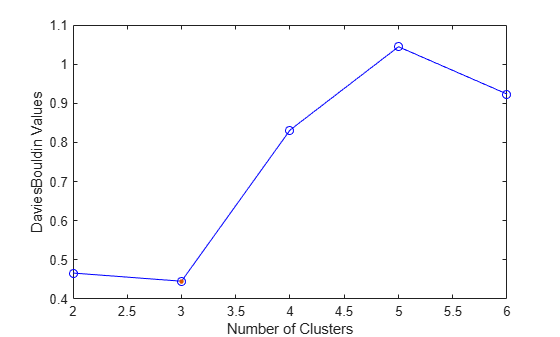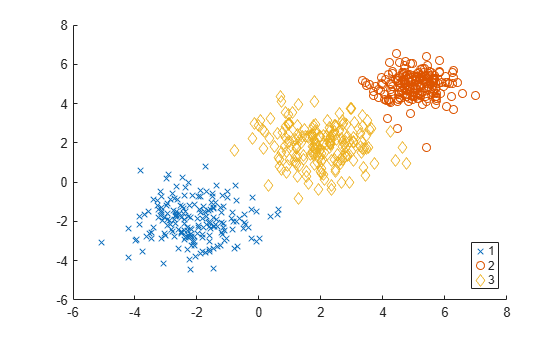# DaviesBouldinEvaluation

Davies-Bouldin criterion clustering evaluation object

## Description

`DaviesBouldinEvaluation` is an object consisting of sample data (`X`), clustering data (`OptimalY`), and Davies-Bouldin criterion values (`CriterionValues`) used to evaluate the optimal number of clusters (`OptimalK`). The Davies-Bouldin criterion is based on a ratio of within-cluster and between-cluster distances. The optimal clustering solution has the smallest Davies-Bouldin index value. For more information, see Davies-Bouldin Criterion.

## Creation

Create a Davies-Bouldin criterion clustering evaluation object by using the `evalclusters` function and specifying the criterion as `"DaviesBouldin"`.

You can then use `compact` to create a compact version of the Davies-Bouldin criterion clustering evaluation object. The function removes the contents of the properties `X`, `OptimalY`, and `Missing`.

## Properties

expand all

### Clustering Evaluation Properties

Clustering algorithm used to cluster the sample data, returned as `'kmeans'`, `'linkage'`, `'gmdistribution'`, or a function handle. If you specify the clustering solutions as an input argument to `evalclusters` when you create the clustering evaluation object, then `ClusteringFunction` is empty.

ValueDescription
`'kmeans'`Cluster the data in `X` using the `kmeans` clustering algorithm, with `EmptyAction` set to `"singleton"` and `Replicates` set to `5`.
`'linkage'`Cluster the data in `X` using the `clusterdata` agglomerative clustering algorithm, with `Linkage` set to `"ward"`.
`'gmdistribution'`Cluster the data in `X` using the `gmdistribution` Gaussian mixture distribution algorithm, with `SharedCov` set to `true` and `Replicates` set to `5`.

Data Types: `double` | `char` | `function_handle`

Name of the criterion used for clustering evaluation, returned as `'DaviesBouldin'`.

Criterion values, returned as a numeric vector. Each value corresponds to a proposed number of clusters in `InspectedK`.

Data Types: `double`

List of the number of proposed clusters for which to compute criterion values, returned as a positive integer vector.

Data Types: `double`

Optimal number of clusters, returned as a positive integer scalar.

Data Types: `double`

Optimal clustering solution corresponding to `OptimalK`, returned as a positive integer column vector. Each row of `OptimalY` represents the cluster index of the corresponding observation (or row) in `X`. If you specify the clustering solutions as an input argument to `evalclusters` when you create the clustering evaluation object, or if the clustering evaluation object is compact (see `compact`), then `OptimalY` is empty.

Data Types: `double`

### Sample Data Properties

Excluded data, returned as a logical column vector. If an element of `Missing` is `true`, then the corresponding observation (or row) in the data matrix `X` is not used in the clustering solutions. If the clustering evaluation object is compact (see `compact`), then `Missing` is empty.

Data Types: `double` | `logical`

Number of observations in the data matrix `X`, ignoring observations with missing (`NaN`) values, returned as a positive integer scalar.

Data Types: `double`

Data used for clustering, returned as a numeric matrix. Rows correspond to observations, and columns correspond to variables. If the clustering evaluation object is compact (see `compact`), then `X` is empty.

Data Types: `single` | `double`

## Object Functions

 `addK` Evaluate additional numbers of clusters `compact` Compact clustering evaluation object `plot` Plot clustering evaluation object criterion values

## Examples

collapse all

Evaluate the optimal number of clusters using the Davies-Bouldin clustering evaluation criterion.

Generate sample data containing random numbers from three multivariate distributions with different parameter values.

```rng("default") % For reproducibility n = 200; mu1 = [2 2]; sigma1 = [0.9 -0.0255; -0.0255 0.9]; mu2 = [5 5]; sigma2 = [0.5 0; 0 0.3]; mu3 = [-2 -2]; sigma3 = [1 0; 0 0.9]; X = [mvnrnd(mu1,sigma1,n); ... mvnrnd(mu2,sigma2,n); ... mvnrnd(mu3,sigma3,n)];```

Evaluate the optimal number of clusters using the Davies-Bouldin criterion. Cluster the data using `kmeans`.

`evaluation = evalclusters(X,"kmeans","DaviesBouldin","KList",1:6)`
```evaluation = DaviesBouldinEvaluation with properties: NumObservations: 600 InspectedK: [1 2 3 4 5 6] CriterionValues: [NaN 0.4663 0.4454 0.8316 1.0444 0.9236] OptimalK: 3 ```

The `OptimalK` value indicates that, based on the Davies-Bouldin criterion, the optimal number of clusters is three.

Plot the Davies-Bouldin criterion values for each number of clusters tested.

`plot(evaluation)`The plot shows that the lowest Davies-Bouldin value occurs at three clusters, suggesting that the optimal number of clusters is three.

Create a grouped scatter plot to visually examine the suggested clusters.

```clusters = evaluation.OptimalY; gscatter(X(:,1),X(:,2),clusters,[],"xod")```The plot shows three distinct clusters within the data: cluster 1 in the lower-left corner, cluster 2 in the upper-right corner, and cluster 3 near the center of the plot.